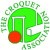# Optimising the ranking system

by Louis Nel
26th February 2005 (Other News)

I have long been looking for a satisfactory numerical estimate for the efficiency of our World Ranking system. Now at last I have found one, namely, the Percentage of Correct Predictions (PCP) over a period.

It is hard to imagine a statistic more natural or more easy to measure or more easy to understand. I was looking at complicated things while this elegant concept was staring me in the face all the time ...

In this study I used the period spanned by the calendar years 2002, 2003 and 2004. For the purpose of determining PCP I took into account only games in which both opponents already had 30 games recorded. That was towards creating a level playing field when a new trial system is to be compared with the current system (I considered 30 games per player to be a reasonable warm-up for a new system). This restriction reduced the total of 43537 games used for ranking purposes to 20806 Test Games for PCP purposes. That is still a substantial sample.

The current ranking system can predict a game result on the basis of either the higher Grade or the higher Index of the players. Since the Grade is officially our ultimate ranking statistic, it is presumably believed to be the better one. I found them to be virtually equally good predictors. Out of the Test Game Total of 20806,

the Grade gave 14185 correct predictions
the Index gave 14192 correct predictions
Index PCP = 68.21

Is a predictive success of 68% good or bad? That depends on the disparities between opponents. If in every game the Winning Probability was 55%, then PCP could be expected to approximate 55%. So to put 68% into perspective, we need the Average Winning Probability. We can never know it exactly, but we can at least estimate it via the formula that translates an Index difference of X points into a Winning Probability of 1/(1 + 10^(-X/500)). To this end I calculated the Average Winning Probability (AWP) for 9 different Indexes (of rival systems under consideration). They gave very similar values. I took the average and so arrived finally at the value AWP = 70.52. The difference AWP - PCP = 2.34 (for Grade) and 2.31 (for Index) provides an estimate of how much the system falls short of the ideal. Mindful that a system derives its knowledge of players only indirectly through game results and moreover does that in the face of randomness and a chronic shortage of data, these numbers leave me with the impression that our ranking system has served us very well.

Nevertheless, the question remains: can it serve us even better? The concept PCP allows objective numerical comparison of systems as regards their predictive success. I investigated how PCP varies with Step-size (recall that Step-size is the maximum Index-increase in one game).The following table summarises what I found.

TABLE: How PCP varies with Step-size
S-s PCP
12 67.45
15 67.84
18 68.15
21 68.24
24 68.33
27 68.35
30 68.39 (optimal value)
33 68.36
36 68.35
39 68.34
42 68.24
45 68.22
48 68.13

Step-size 60 (largest currently in use, not in the table) gives a PCP = 68.01.

For the optimal step-size 30 the introduction of a Grade worsens predictive efficiency. Small Smoothing Parameters (0.5 or lower) have virtually no effect. SP = 0.9 causes a notable decline; SP = 0.97 (largest value employed by the current system) causes a precipitous decline. This is not surprising when one considers that the larger the SP the more it looks at past form at the expense of current form and the latter is surely more relevant for prediction.

In summary, the two key parameters that govern predictive success, have the following optimal values:

Step-size = 30 accompanied by Smoothing Parameter = 0.

Let I30 denote the system consisting of an Index with this optimal step-size of 30 and no Grade (that is the effect of having SP = 0).

PCP Comparison Table
System PCP
CG 68.18
CI 68.21
I30 68.39

The improvement in predictive efficiency promised by I30 may seem marginal rather than substantial. However, there are additional advantages worthy of consideration, as follows.

Our current system is so complicated that few people understand it. Even those, like myself, that do understand it well enough to do the calculations, cannot claim to fully understand all the side effects of its large and variable Smoothing Parameter. Human beings are not walking computers. The presumed purpose of the Grade is to mollify the more volatile Index. However, as soon as the Grade and Index are far apart -- which seems like precisely the kind of circumstance in which the Grade should prove its worth -- we find the Grade acting up with anomalous behaviour. Namely, as is well known, a player's Grade may increase even if the next game is lost or decrease even if the next game is won. This misbehaviour, together with the inherent complexity, contributes to scepticism in the public mind.

By contrast, I30 would be, by comparison, delightfully simple to understand. It hinges on just two things:

(1) After each game the Winner's Index is increased and the Loser's Index is decreased by the same amount, called the Adjustment.

(2) That Adjustment = Step-size * (Loser's winning probability)

Even without a calculator or tables, anybody could form a good idea of each Index adjustment that arises. For example, suppose you are playing a game in which you estimate your Winning Probability to be 1/3 (thereby, automatically you are estimating your opponent's WP to be 2/3). Then if you lose, the estimated Adjustment is 30 1/3 = 10 points. if you win, the estimated Adjustment is 30 2/3 = 20 points.

Everybody willing to make a little effort could understand how this works. When you afterwards see on Chris' website that the actual Adjustment turned out to be 12 points, you can use the equation